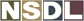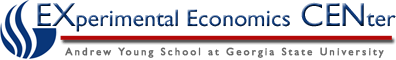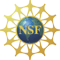Students / Subjects# GDP Deflator

Essentially, GDP Deflator is an adjustment for the impact of changes in prices on changes in nominal GDP. GDP Deflator can be considered the most comprehensive measure of inflation since a wide array of goods and services are included in its construction. But it may not reflect the full impact of inflation on consumer welfare because it does not include imported goods and services that constitute a significant portion of what people buy.

GDP Deflator is the ratio of the value of aggregate final output at current market prices (Nominal GDP) to its value at the base year prices (Real GDP). In effect the basket of goods for the construction of this price index includes all the final output produced within the geographic boundaries of the country.

GDP Deflator = Nominal GDP/ Real GDP

### Nominal GDP:

Nominal GDP can change from time to time because of two reasons:

• changes in the physical volume of output or
• changes in the prices at which output is valued

We want to use GDP to look at changes in the physical volume of output. Since Nominal GDP can also change due to changes in the prices at which output is valued it is necessary to "deflate" the value recorded for Nominal GDP (GDP with inflation) into "real" dollars so we can make comparisons across years.

### Real GDP:

When we divide GDP at current market prices (Nominal GDP) by the corresponding GDP deflator, we obtain what is called real GDP.

Real GDP = Nominal GDP / GDP Deflator

Real GDP can change only because of changes in the physical volume of output. As a result Real GDP is considered a better measure of economic growth than nominal GDP.

### Adjustments to GDP with GDP Deflator

Since inflation changes from year to year, and a nations productivity level over time is tracked in monetary terms using GDP, how can you tell if a change in a country's level of output is due to a real change in productivity or whether it is due to fluctuations in the level of the prices of that output? The answer is you can't.

If you recall the concept of "holding all else constant" you will quickly realize that without adjusting GDP to account for the effect of inflation, you will be unable to accurately compare a country's GDP from year to year.

To deal with this issue, a "fudge factor" called the GDP deflator is used to convert Nominal GDP (GDP with the effects of inflation) into Real GDP (GDP without the effects of inflation). Nominal GDP is divided by the GDP deflator to get Real GDP. Basically, the GDP deflator is used to "cancel out" the effects of inflation.

Back to National Income Accounting

Back to Inflation